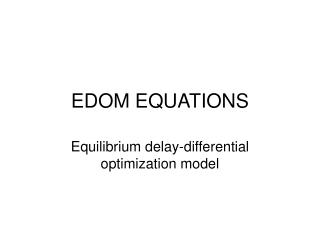DownloadDownload PresentationEDOM EQUATIONS

# EDOM EQUATIONS

Télécharger la présentation## EDOM EQUATIONS

- - - - - - - - - - - - - - - - - - - - - - - - - - - E N D - - - - - - - - - - - - - - - - - - - - - - - - - - -
##### Presentation Transcript

1. EDOM EQUATIONS Equilibrium delay-differential optimization model

2. Biomass and numbers EACH PREDICTION IS A RATIO OF GAIN RATE TO LOSS RATE

3. Larval settlement

4. Recruitment Where: a=KRo/Lo b=(K-1)/Lo and Lo≈Ro(wr+kw∞/M)/(M+k)

5. Effort (fishing mortality) distribution HERE, Wi IS THE “GRAVITY WEIGHT” Wi=Bip/Ci

6. Test parameter values

7. Derived parameters